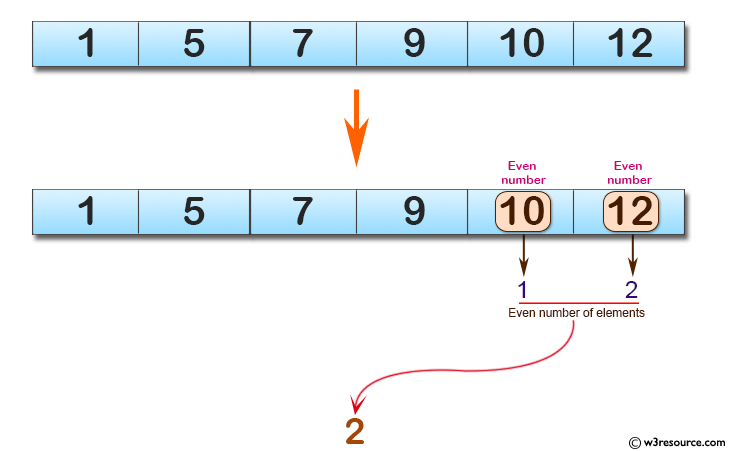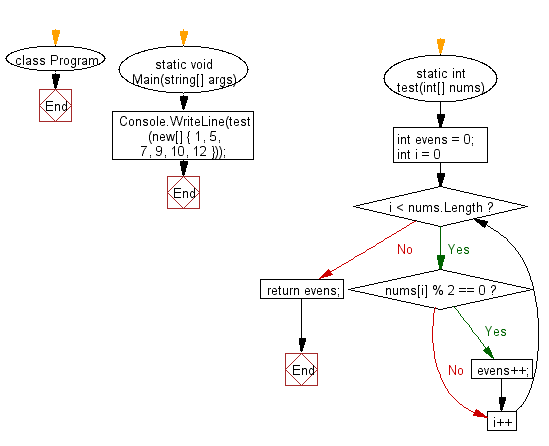﻿ C# - Count the number of even elements in a array# C# Sharp Basic Algorithm Exercises: Count the number of even elements in a given array of integers

## C# Sharp Basic Algorithm: Exercise-109 with Solution

Write a C# Sharp program to count the number of even elements in a given array of integers.

Pictorial Presentation:Sample Solution:-

C# Sharp Code:

``````using System;
namespace exercises
{
class Program
{
static void Main(string[] args)
{
Console.WriteLine(test(new[] { 1, 5, 7, 9, 10, 12 }));
}
static int test(int[] nums)
{
int evens = 0;

for (int i = 0; i < nums.Length; i++)
{
if (nums[i] % 2 == 0) evens++;
}
return evens;
}
}
}
```
```

Sample Output:

`2`

Flowchart:C# Sharp Code Editor:

Improve this sample solution and post your code through Disqus

What is the difficulty level of this exercise?

Test your Programming skills with w3resource's quiz.

﻿